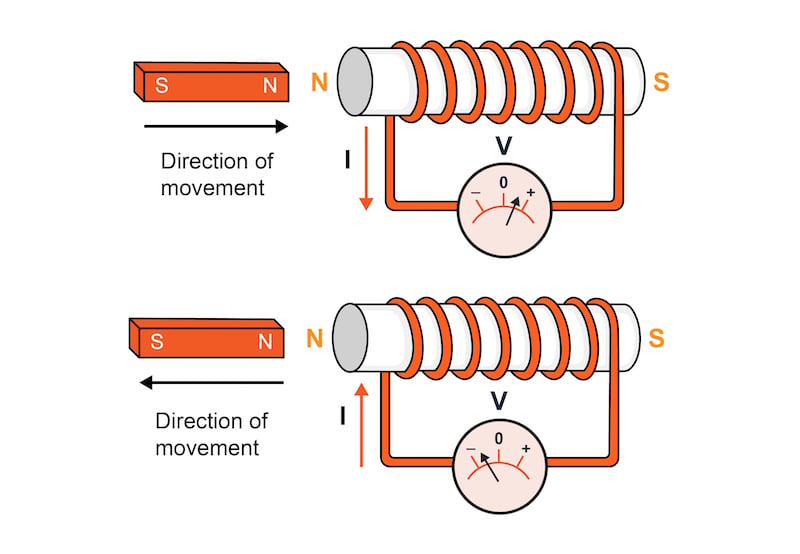# Lenz’s Law and Faraday’s Law Calculator

## This calculator uses Faraday's and Lenz's laws to calculate the magnitude and polarity of the induced electromotive force (EMF) caused by a change in magnetic flux through a closed-loop coil.

The application of this calculator is when trying to understand the force and voltage generated by a changing magnetic field around a closed loop. You can enter any three of the four variables:

• The number of turns of a coil
• The change in magnetic flux
• The time period for the change
• The EMF

From there, the calculator will return the result for the fourth variable.

### Faraday's Law Equation and Definition

Faraday’s law of electromagnetic induction states that any change in a magnetic field will induce an electromotive force in a conductive coil that is directly proportional to the rate of change in the inducing magnetic field. This EMF, measured in volts, will also create a current flow. Faraday’s equation only tells us the magnitude of the EMF, not the polarity:

$$\epsilon = N \frac{d\theta}{dt}$$

Where:

• $$\epsilon$$ = the electromotive force, in volts
• N = the number of turns in a coil of wire
• $$d\theta$$ = the change in magnetic flux, in webers
• $$dt$$ = the time over which the change in flux occurred, in seconds

### Lenz’s Law Equation and Definition

To determine the polarity of the induced EMF, we must turn to Lenz’s law which states that when a changing magnetic field induces a current in a conducting coil, the induced current will generate a magnetic field that opposes the inducing magnetic field. To put it simply, the induced current opposes the changing magnetic flux which is producing it, as shown in Figure 1.##### Figure 1. Faraday’s law and Lenz’s law define the induced EMF generated in a coil by a changing magnetic field

When the north pole of the magnet in the figure above moves closer to or further from the loop, an EMF will be created with polarity such that the induced current in the loop will create a magnetic field that opposes the changing magnetic field from the magnet.

Putting together Faraday’s equation (magnitude) and Lenz’s equation (polarity), we get the following:

$$\epsilon = -N \frac{d\theta}{dt}$$

### Alternate Naming Conventions—Faraday-Lenz Law and More

Because Faraday’s law, Lenz’s Law, and Maxwell’s equations are so closely related to each other, you may see other names used for these laws including:

• Faraday’s law of electromagnetic induction